Histograms & Box Plots Math Videos for Kids - 6th, 7th, & 8th Grades
1%
It was processed successfully!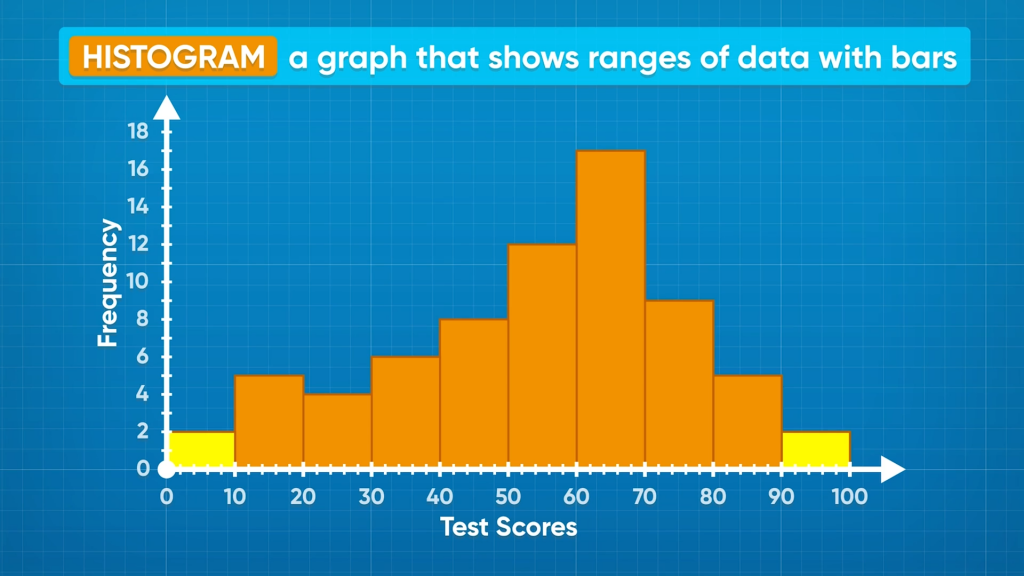WHAT ARE HISTOGRAMS AND BOX PLOTS?

Histograms are a special kind of bar graph that shows a bar for a range of data values instead of a single value. A box plot is a data display that draws a box over a number line to show the interquartile range of the data. The ‘whiskers’ of a box plot show the least and greatest values in the data set.

To better understand histograms & box plots…

WHAT ARE HISTOGRAMS AND BOX PLOTS?. Histograms are a special kind of bar graph that shows a bar for a range of data values instead of a single value. A box plot is a data display that draws a box over a number line to show the interquartile range of the data. The ‘whiskers’ of a box plot show the least and greatest values in the data set. To better understand histograms & box plots…

## LET’S BREAK IT DOWN!

### What are histograms?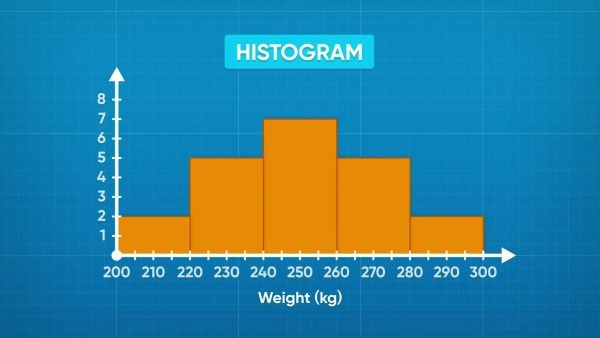If a data set has a lot of different measurements, displaying it using line graphs does not always help to interpret the information. Instead, you can display the data using a type of bar graph called a histogram. In a histogram, bars represent ranges instead of individual values. These bars are called bins, and they are presented continuously with no spaces between them. Each bar represents a range of data points, and the height of the bar tells us how many data points are in that range. Now you try: Find a picture of a histogram online or in your textbook. Identify the bins and the height of each bar.

What are histograms? If a data set has a lot of different measurements, displaying it using line graphs does not always help to interpret the information. Instead, you can display the data using a type of bar graph called a histogram. In a histogram, bars represent ranges instead of individual values. These bars are called bins, and they are presented continuously with no spaces between them. Each bar represents a range of data points, and the height of the bar tells us how many data points are in that range. Now you try: Find a picture of a histogram online or in your textbook. Identify the bins and the height of each bar.

### What can we learn from reading a histogram?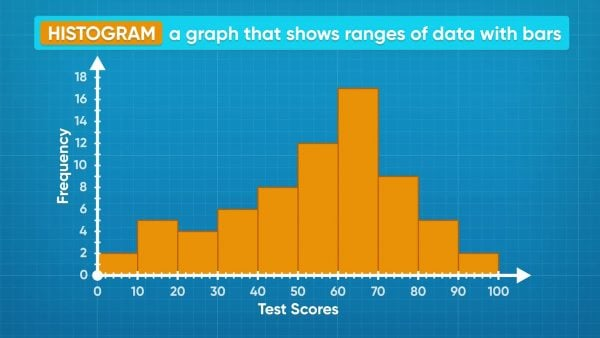On a test, the students on your class got the following scores: 58, 55, 58, 55, 59, 56, 54, 62, 66, 62, 62, 61, 68, 70, 66, 70, 69, 66, 63, 70, 66, 66, 62, 61, 76, 77, 71, 75, 71, 79, 79, 78, 85, 88, 85, 81, 85, 86, 95, 99, 91, 95. You can separate the data into ranges. Here, it makes sense to choose ranges 50-59, 60-69, 70-79, 80-89, and 90-99. You want to have enough bins to make several distinct ranges, but not so many that they are hard to interpret. If you look at the histogram represented by the data, you can see that the most common grades are in the 60-69% range. The least common grades are in the 90-100% range. Those are called outliers. Now you try: Find a picture of a histogram online or in your textbook. Which bin contained the least number of values? Where there any outliers?

What can we learn from reading a histogram? On a test, the students on your class got the following scores: 58, 55, 58, 55, 59, 56, 54, 62, 66, 62, 62, 61, 68, 70, 66, 70, 69, 66, 63, 70, 66, 66, 62, 61, 76, 77, 71, 75, 71, 79, 79, 78, 85, 88, 85, 81, 85, 86, 95, 99, 91, 95. You can separate the data into ranges. Here, it makes sense to choose ranges 50-59, 60-69, 70-79, 80-89, and 90-99. You want to have enough bins to make several distinct ranges, but not so many that they are hard to interpret. If you look at the histogram represented by the data, you can see that the most common grades are in the 60-69% range. The least common grades are in the 90-100% range. Those are called outliers. Now you try: Find a picture of a histogram online or in your textbook. Which bin contained the least number of values? Where there any outliers?

### How can we organize our data for a histogram?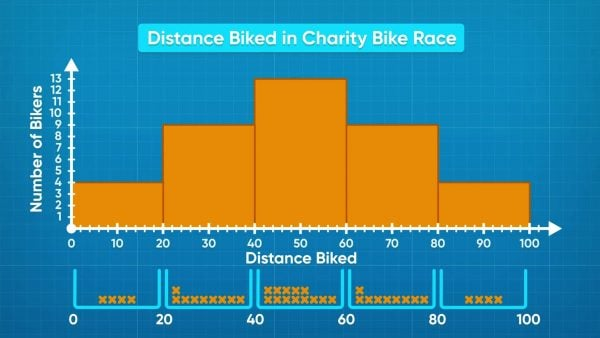When you have a lot of data, you first have to decide how many bins you would like to use, and what the range of each bin should be. In a bike race, the distances in kilometers that cyclists rode are: 5, 8.25, 15.5, 18, 20, 22.5, 28, 28, 29.5, 30, 30, 36.5, 38, 42.5, 45.75, 46, 47, 48, 48, 50, 50, 52, 55, 58, 58, 59, 63.25, 65.5, 67, 70, 70, 72, 75, 75, 76, 83, 87.75, 94.5, 95. You can organize the data into 5 bins, the first one 0-19, then 20-39, 40-59, 60-79, and 80-99. You can then draw the axes for the graph and label the bin sizes at the bottom along the x-axis, and label it “Distance Biked.” The y-axis can be labeled “Number of Cyclists.” Now as you read the data, you can make a tick to count each time a point contributes to a bin. The 0-19 bin has a frequency of 4, 20-39 has 9, 40-59 has 13, 60-79 has 9, and 80-99 has 4. You can see that the most common distance biked is 40-59 kilometers! If you choose bin size 5, you would have a lot more bars and they would be harder to interpret. If you choose bin size 40, you would only have 3 bars, which is not clear either. It is important to choose a bin size that helps you make sense of the data. Now you try: A data set contains the following data: 12, 18, 19, 5, 17, 10, 3, 2, 24, 1, 22. What size bins would you choose for your histogram?

How can we organize our data for a histogram? When you have a lot of data, you first have to decide how many bins you would like to use, and what the range of each bin should be. In a bike race, the distances in kilometers that cyclists rode are: 5, 8.25, 15.5, 18, 20, 22.5, 28, 28, 29.5, 30, 30, 36.5, 38, 42.5, 45.75, 46, 47, 48, 48, 50, 50, 52, 55, 58, 58, 59, 63.25, 65.5, 67, 70, 70, 72, 75, 75, 76, 83, 87.75, 94.5, 95. You can organize the data into 5 bins, the first one 0-19, then 20-39, 40-59, 60-79, and 80-99. You can then draw the axes for the graph and label the bin sizes at the bottom along the x-axis, and label it “Distance Biked.” The y-axis can be labeled “Number of Cyclists.” Now as you read the data, you can make a tick to count each time a point contributes to a bin. The 0-19 bin has a frequency of 4, 20-39 has 9, 40-59 has 13, 60-79 has 9, and 80-99 has 4. You can see that the most common distance biked is 40-59 kilometers! If you choose bin size 5, you would have a lot more bars and they would be harder to interpret. If you choose bin size 40, you would only have 3 bars, which is not clear either. It is important to choose a bin size that helps you make sense of the data. Now you try: A data set contains the following data: 12, 18, 19, 5, 17, 10, 3, 2, 24, 1, 22. What size bins would you choose for your histogram?

### What are box plots?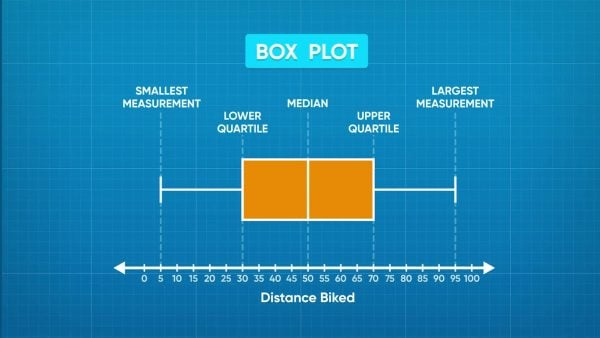Histograms are great for showing what data ranges are most and least common, but they do not tell details like the range or the median. You can use box plots to present these values. They have 5 vertical lines. The lines farthest on the left and right tell the least and greatest values of the data set. The line in the middle is the median. The other two lines are called the lower quartile and upper quartile. The lower quartile line is on the left of the median, and it tells us that one-quarter of the data points are less than or equal to the lower quartile. The upper quartile is on the right of the median and tells us that one-quarter of the data points are greatest than or equal to the upper quartile. Now you try: Find an image of a box plot online or in your textbook. Identify the 5 key values in the box plot.

What are box plots? Histograms are great for showing what data ranges are most and least common, but they do not tell details like the range or the median. You can use box plots to present these values. They have 5 vertical lines. The lines farthest on the left and right tell the least and greatest values of the data set. The line in the middle is the median. The other two lines are called the lower quartile and upper quartile. The lower quartile line is on the left of the median, and it tells us that one-quarter of the data points are less than or equal to the lower quartile. The upper quartile is on the right of the median and tells us that one-quarter of the data points are greatest than or equal to the upper quartile. Now you try: Find an image of a box plot online or in your textbook. Identify the 5 key values in the box plot.

### How do we make a box plot?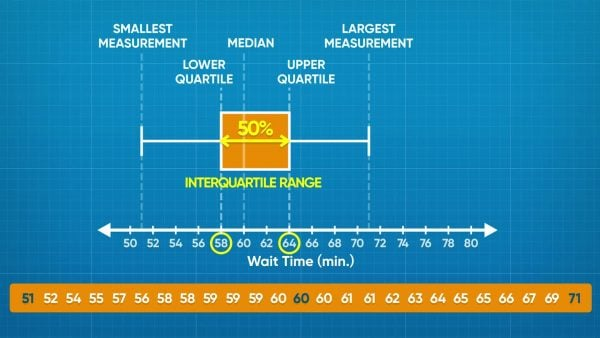The wait times for a rollercoaster, in minutes, are: 51, 54, 55, 56, 57, 57, 58, 58, 58, 59, 59, 59, 59, 59, 60, 61, 61, 61, 61, 62, 62, 64, 64, 66, 67, 69, 70, 71, 71. To start making the box plot, locate the least and greatest values. Luckily, this data set is already sorted from least to greatest, so you can see that these values are 51 and 71. Place and label these values on the plot. Next, find the median. The number in the middle is 60. That means half of the people waited greater than or equal to 60 minutes, and half the people waited less than or equal to 60 minutes. To find the lower quartile, we find the time that is the middle data point in the range 51 to 60, and to find the upper quartile we find the middle data point in the range 60 to 71. The lower quartile is 58 and the upper quartile is 64. If 25% of people waited 58 minutes or less, and 25% of people waited 64 minutes or more, that means that at least half of the people waited between 58 and 64 minutes. This is called the interquartile range. Now you try: A set of data has a range of 3 to 85. The median is 72. The lower and upper quartiles are 35 and 78. Draw a box plot using this information.

How do we make a box plot? The wait times for a rollercoaster, in minutes, are: 51, 54, 55, 56, 57, 57, 58, 58, 58, 59, 59, 59, 59, 59, 60, 61, 61, 61, 61, 62, 62, 64, 64, 66, 67, 69, 70, 71, 71. To start making the box plot, locate the least and greatest values. Luckily, this data set is already sorted from least to greatest, so you can see that these values are 51 and 71. Place and label these values on the plot. Next, find the median. The number in the middle is 60. That means half of the people waited greater than or equal to 60 minutes, and half the people waited less than or equal to 60 minutes. To find the lower quartile, we find the time that is the middle data point in the range 51 to 60, and to find the upper quartile we find the middle data point in the range 60 to 71. The lower quartile is 58 and the upper quartile is 64. If 25% of people waited 58 minutes or less, and 25% of people waited 64 minutes or more, that means that at least half of the people waited between 58 and 64 minutes. This is called the interquartile range. Now you try: A set of data has a range of 3 to 85. The median is 72. The lower and upper quartiles are 35 and 78. Draw a box plot using this information.

## HISTOGRAMS & BOX PLOTS VOCABULARY

Line plot
A plot that gathers data on a number line.
Bins
Equally-spaced intervals that we use to sort data on a graph.
Bar graph
A graph that represents data using vertical or horizontal bars.
Histogram
A type of bar graph that displays ranges of data.
y-axis
The vertical axis. In histograms, it shows the number of values in a range.
x-axis
The horizontal axis. In histograms, it shows the range of measurements for each bin.
Data sets or points that are not common and far away from the rest of the data set.
The number in the middle of a sorted data set.
A value, to the left of which 25% of the data lies.
A value, to the right of which 25% of the data lies.
50% of the data, which lies between the lower and upper quartiles.

## HISTOGRAMS & BOX PLOTS DISCUSSION QUESTIONS

### In a graph, what are outliers?

Outliers are data points that are not common and far away from the range with the greatest frequency.

### A histogram has a bar from 0-20 with height 4, a bar from 20-40 with height 2, a bar of 40-60 with height 7, and a bar of 60-80 with height 4. The plot represents the ages of people at a wedding. What can you learn from the graph?

The largest number of people are in the 40-60 range. The least number of people are between 20 and 40. Maybe the people who got married are in their 40s, and they or their friends have some children.

### How could you change this graph to get more information from it?

I could increase the number of bins, and then I might know more about the ages of people in attendance.

### What do box plots tell you that histograms don’t?

Box plots tell me the least and greatest value in the data, as well as the median. They also tell the lower and upper quartile. Those values can only be estimated from histograms.

### How do you calculate the lower and upper quartile?

First, I find the median by counting data values from the left and right sides toward the middle until I reach one or two numbers in the middle. To find the lower quartile, I do the same thing between the least value and the median, and to find the upper quartile, I find the middle between the median and the greatest value. It makes sense because the median cuts the data in half, and if I cut it in half one more time, I get quarters!
X

## Success

We’ve sent you an email with instructions how to reset your password.
Ok